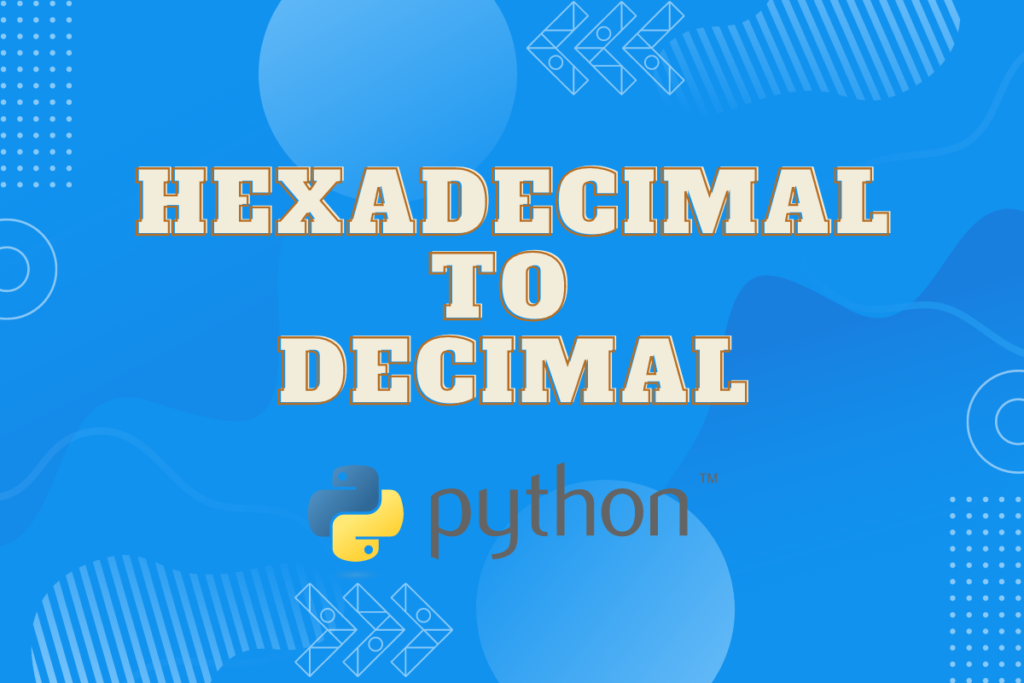# Converting Hex to Decimal in Python Real Quick!Data can be recorded in umpteen formats. There is no universal format which serves as a one size fits all, for data recording. Depending on the purpose or the source from which the data is collected, the format which is used to record the data highly varies. But, when it comes to data analysis, one faces the task of harmonising the data from different formats.

Homogeneity in format is crucial for analysing data & this article shall detail the data conversion techniques in Python to establish this homogeneity. To be more specific, this article shall deal with the conversion of data from hexadecimal to decimals using Python.

Any of the following methods which best suits the purpose shall be deployed for the conversion.

• Using int( ) function
• Using literal_eval( ) function
• Using the for loop
• Using the while loop

## Method I – Using int( ) function

This method makes use of int( ), an in-built function within Python for converting the given hexadecimal data into decimal format. The following code demonstrates how the int( ) function works. It is imperative that one provides the base 16 following the input variable to instruct the int( ) function for converting it into hexadecimal.

```hex = '75FC'
dec = int(hex, 16)
print ('Input hexadecimal value:', hex, '\n', 'Converted decimal value:', dec)
```

The following result is displayed when the above code is run.

## Method II – Using literal_eval( ) function

For those who do not desire to feed in the base value within the int( ) function and would like the function itself to take care of all the conversion, then the literal_eval( ) function might just be the thing you are looking for!

This function never asks for anything but the input from which it predicts the format of the data and converts it into its equivalent decimal version. It is to be noted that this function lies within the ast library. So, it only works after being imported!

The following code demonstrates how this function works.

```from ast import literal_eval
hex = '0x75FC'
dec = literal_eval(hex)
print ('Input hexadecimal value:', hex, '\n', 'Converted decimal value:', dec)
```

## Method III – Using the for loop

For those who are keen on flexing their coding skills without relying on the built-in functions of Python, the hexadecimal to decimal conversion might as well be done using the for loop. But this technique comes with a pre-requisite!

One ought to create a dictionary containing the decimal equivalents of all the hexadecimal such as the one given below. It is only with this information that for loop can compare against the given input and return the result after conversion.

```Ref = {'0':0, '1':1, '2':2, '3':3, '4':4, '5':5, '6':6, '7':7, '8':8, '9':9,
'A':10, 'B':11, 'C':12, 'D':13, 'E':14, 'F':15}
```

Once done, let’s assign a variable ‘r’ to zero & assign the maximum power value from the input to another variable ‘s’.

```r = 0
s = len(hex)-1
```

Now a for loop shall be constructed with the variable ‘r’ raised to the power of ‘s’ & ‘s’ decremented by ‘1’ in each run as shown below.

```for num in hex:
r = r+Ref[num]*16**s
s = s-1
```

Following is the result when the above code is run.

## Method IV – Using the while loop

There is another way of converting hexadecimal data to decimal without using the built-in functions of Python & this time it is through the while loop. Let’s now take input rather than feeding in the data & convert it into decimal using the below code.

```hex = input("Input hexadecimal value: ") .strip( ).upper( )
```

The strip( ) and upper( ) functions are used to remove the spaces and convert the alphabets into uppercase from the given input. Then, it is run through the while loop for conversion.

```l = count = i = 0
s = len(hex)-1
while s>=0:
if hex[s]>='0' and hex[s]<='9':
r = int(hex[s])
elif hex[s]>='A' and hex[s]<='F':
r = ord(hex[s])-55
else:
l = 1
break
count = count+(r*16**i)
s = s-1
i = i+1
if l == 0:
print('Converted decimal value:', count)
else:
print('Incorrect input')
```